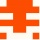Home
IT Knowledge
Inspiration
Languages
EN

# JavaScript - average value using array reduce()

0 points
Created by:Lilly-Grace-Greig
211

In this article, we would like to show you how to calculate the average value with `reduce()` method in JavaScript.

In below example, we pass an arrow function calculating the average value of `numbers` to the`reduce()` method.

Runnable example:

``````// ONLINE-RUNNER:browser;

const numbers = [10, 20];

function average(array) {
return array.reduce((a, b) => (a + b)) / array.length;
}

console.log(average(numbers)); //15``````